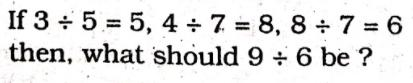## Symbols & Notations TYPE-I (vi) Questions based on figures & mathematical operations

• Post author:
• Post category:Blog

TYPE-I (vi)

1. Some equations are solved on the basis of a certain system. Find out the correct answer for the unsolved equation on that basis. If 8 + 8 = 72, 5 + 5 = 30 and 7 + 7 = 56, what is 6 + 6 = ?

(1) 40

(2) 42

(3) 30

(4) 36

(FCI Assistant Grade-II Exam. 22.01.2012 Paper-I)

2. Some equations are solved on the basis of a certain system. Find out the correct answer for the unsolved equation on that basis.(1) 4

(2) 9

(3) 5

(4) 6

FCI Assistant Grade-III Exam. 25.02.2012 (Paper-I) North Zone (Ist Sitting)

3. Some equations are solved on the basis of a certain system. On the same basis find out the correct answer for the unsolved equation. If

8 x 2 = 61; 8 x 5 = 04, what is 8 x 10 = ?

(1) 80

(2) 08

(3) 8

(4) 0

FCÍ Assistant Grade-III Exam. 05.02.2012 (Paper-I) East Zone (IInd Sitting)

4. Some equations are solved on the basis of a certain system. Find the correct answer for the unsolved equation on that basis.

5 + 7 + 2 = 725, 6 + 9 + 0 = 906, 8 + 4 + 3 = ?

(1) 815

(2) 384

(3) 438

(4) 834

(SSC Multi-Tasking (Non-Tech.) Staff Exam. 16.02.2014)

5. Some equations are solved on the basis of a certain system. Find the correct answer for the unsolved equation on that basis.

678 = 366, 567 = 255, 946 = ?

(1) 334

(2) 499

(3) 699

(4) 634

(SSC Multi-Tasking (Non-Tech.) Staff : Exam. 23.02.2014, ļInd Sitting)